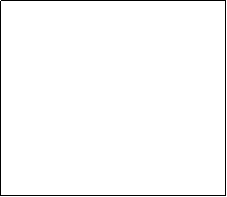Area of a Square              Area of a Rectangle              Area of a TriangleAREA of a SQUARE = the SQUARE of one side A = s2 Find the area for the following squares: 1) A square with a side of 6m: A=36 m2   2) A square with a side of 12 ft   A=144ft2               3) A square with a side of x   xin2